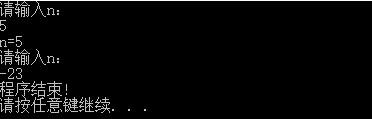## c++中的break,continue,goto语句

2019-09-15 16:38  myself914  阅读(1274)  评论(0编辑  收藏  举报

1、用法：

2、实例：

#include "stdafx.h"
#include<iostream>
using namespace std;
int main()
{
int i, n;
for (i = 1; i <= 3; i++)
{
cout << "请输入n：" << endl;
cin >> n;
if (n < 0)
break;
cout << "n=" << n << endl;
}
cout << "程序结束!" << endl;
return 0;

}



3、运行结果

1、用法：

2、实例：


#include<iostream>
using namespace std;
int main()
{
int i, n;
for (i = 1; i <= 3; i++)
{
cout << "请输入n：" << endl;
cin >> n;
if (n < 0)
goto end;
cout << "n=" << n << endl;
}
end:cout << "程序结束!" << endl;
return 0;
}


3运行结果：braek与goto：

1、用法：

2、实例：

#include<iostream>
using namespace std;
int main()
{
int i, n;
for (i = 1; i <= 3; i++)
{
cout << "请输入n：" << endl;
cin >> n;
if (n < 0)
continue;
cout << "n=" << n << endl;
}
cout << "程序结束!" << endl;
return 0;
}


3、运行结果：